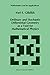Aanmelden
 Aangemeld blijven (i)

De tip van euro-boek.nl
Zoekfuncties
actueel
- 0 resultaten
laagste prijs: € 149,79, hoogste prijs: € 213,50, gemiddelde prijs: € 163,711bestellen
bij Indigo.ca
C\$ 222,95
(ongeveer € 155,27)
bestellen

Ordinary and Stochastic Differential Geometry as a Tool for Mathematical Physics - nieuw boek

ISBN: 9789048147311

The geometrical methods in modem mathematical physics and the developments in Geometry and Global Analysis motivated by physical problems are being intensively worked out in contemporary … Meer...

new in stock. Verzendingskosten:zzgl. Versandkosten., exclusief verzendingskosten
2
bestellen
bij Biblio.co.uk
\$ 258,87
(ongeveer € 213,50)
verzending: € 11,97
bestellen
Ordinary and Stochastic Differential Geometry as a Tool for Mathematical Physics (Mathematics and Its Applications) - pocketboek

2010, ISBN: 9789048147311

gebonden uitgave

LAP LAMBERT Academic Publishing, 2010. New. Statistical Modeling of industrial Problems. This text book presents the application of Nondominated Sorting Genetic Algorithm II (NSGA-II) a… Meer...

IND, GBR - Verzendingskosten: EUR 11.97 A - Z Books, Revaluation Books
3bestellen
bij Amazon.de (Intern. Bücher)
€ 149,79
verzending: € 3,00
bestellen

Ordinary and Stochastic Differential Geometry as a Tool for Mathematical Physics (Mathematics and Its Applications (closed)) (Mathematics and Its Applications (374), Band 374) - pocketboek

2010

ISBN: 9789048147311

Springer, Taschenbuch, Auflage: Softcover reprint of hardcover 1st ed. 1996, 212 Seiten, Publiziert: 2010-12-08T00:00:01Z, Produktgruppe: Buch, Hersteller-Nr.: biography, 0.73 kg, Geometr… Meer...

Verzendingskosten:Die angegebenen Versandkosten können von den tatsächlichen Kosten abweichen. (EUR 3.00)
4bestellen
bij Hugendubel.de
€ 149,99
verzending: € 0,00
bestellen

Ordinary and Stochastic Differential Geometry as a Tool for Mathematical Physics - pocketboek

ISBN: 904814731X

gebonden uitgave

Ordinary and Stochastic Differential Geometry as a Tool for Mathematical Physics ab 149.99 € als Taschenbuch: Softcover reprint of hardcover 1st ed. 1996. Aus dem Bereich: Bücher, Wissens… Meer...

Nr. 13446562. Verzendingskosten:, , DE. (EUR 0.00)
5bestellen
bij Hugendubel.de
€ 149,99
bestellen

Ordinary and Stochastic Differential Geometry as a Tool for Mathematical Physics - pocketboek

ISBN: 904814731X

gebonden uitgave

Ordinary and Stochastic Differential Geometry as a Tool for Mathematical Physics ab 149.99 € als Taschenbuch: Softcover reprint of hardcover 1st ed. 1996. Aus dem Bereich: Bücher, Taschen… Meer...

Nr. 13446562. Verzendingskosten:Kein Versand in Ihr Zielland., exclusief verzendingskosten

Bijzonderheden over het boek
auteur:

Titel:

ISBN:

9789048147311

This book develops new unified methods which lead to results in parts of mathematical physics traditionally considered as being far apart. The emphasis is three-fold: Firstly, this volume unifies three independently developed approaches to stochastic differential equations on manifolds, namely the theory of Itô equations in the form of Belopolskaya-Dalecky, Nelson's construction of the so-called mean derivatives of stochastic processes and the author's construction of stochastic line integrals with Riemannian parallel translation. Secondly, the book includes applications such as the Langevin equation of statistical mechanics. Nelson's stochastic mechanics (a version of quantum mechanics), and the hydrodynamics of viscous incompressible fluid treated with the modern Lagrange formalism. Considering these topics together has become possible following the discovery of their common mathematical nature. Thirdly, the work contains sufficient preliminary and background material from coordinate-free differential geometry and from the theory of stochastic differential equations to make it self-contained and convenient for mathematicians and mathematical physicists not familiar with those branches. Audience: This volume will be of interest to mathematical physicists, and mathematicians whose work involves probability theory, stochastic processes, global analysis, analysis on manifolds or differential geometry, and is recommended for graduate level courses.

Gedetalleerde informatie over het boek. - Ordinary and Stochastic Differential Geometry as a Tool for Mathematical Physics

EAN (ISBN-13): 9789048147311
ISBN (ISBN-10): 904814731X
Gebonden uitgave
pocket book
Verschijningsjaar: 2010
Uitgever: Springer
Gewicht: 0,328 kg
Taal: eng/Englisch

Boek bevindt zich in het datenbestand sinds 2011-06-21T08:41:32+02:00 (Amsterdam)
Detailpagina laatst gewijzigd op 2021-12-05T20:15:03+01:00 (Amsterdam)
ISBN/EAN: 9789048147311

ISBN - alternatieve schrijfwijzen:
90-481-4731-X, 978-90-481-4731-1

Gegevens van de uitgever

Auteur: Yuri E. Gliklikh
Titel: Mathematics and Its Applications; Ordinary and Stochastic Differential Geometry as a Tool for Mathematical Physics
Uitgeverij: Springer; Springer Netherland
Verschijningsjaar: 2010-12-08
Dordrecht; NL
Gedrukt / Gemaakt in
Gewicht: 0,454 kg
Taal: Engels
149,79 € (DE)
153,99 € (AT)
165,50 CHF (CH)
POD

BC; Previously published in hardcover; Hardcover, Softcover / Mathematik/Sonstiges; Angewandte Mathematik; Verstehen; Probability theory; Stochastic processes; differential geometry; manifold; mathematical physics; stochastic process; B; Applications of Mathematics; Probability Theory and Stochastic Processes; Global Analysis and Analysis on Manifolds; Differential Geometry; Applications of Mathematics; Probability Theory; Global Analysis and Analysis on Manifolds; Differential Geometry; Mathematics and Statistics; Wahrscheinlichkeitsrechnung und Statistik; Stochastik; Numerische Mathematik; Differentielle und Riemannsche Geometrie; BB

Introduction. I. Elements of Coordinate-Free Differential Geometry. II. Introduction to Stochastic Analysis in Rn III. Stochastic Differential Equations on Manifolds. IV. Langevin's Equation in Geometric Form. V. Nelson's Stochastic Mechanics. VI. The Lagrangian Approach to Hydrodynamics. Appendix: Solution of the Newton-Nelson Equation with Random Initial Data; Yu.E. Glikhlikh, T.J. Zastawniak. References. Index.

Laatste soortgelijke boek:9789401586344 Ordinary and Stochastic Differential Geometry as a Tool for Mathematical Physics (Yuri E. Gliklikh)

< naar Archief...